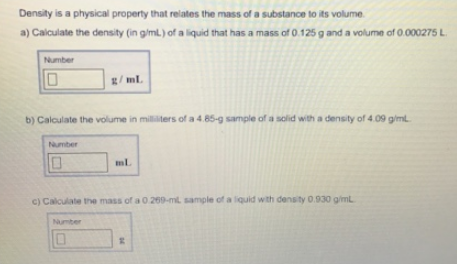# Problem: Density is a physical property that rotates the mass of a substance to its volume. a) Calculate the density (in g/mL) of a liquid that has a mass of 0.125 g and a volume of 0.000275 L. b) Calculate the volume in milliliters of a 4.85-g sample of a solid with a density of 4.09 g/mL. c) Calculate the mass of a 0.269-mL, sample of a liquid with density 0.930 g/mL.

###### FREE Expert Solution
97% (144 ratings)###### Problem Details

Density is a physical property that rotates the mass of a substance to its volume.

a) Calculate the density (in g/mL) of a liquid that has a mass of 0.125 g and a volume of 0.000275 L.

b) Calculate the volume in milliliters of a 4.85-g sample of a solid with a density of 4.09 g/mL.

c) Calculate the mass of a 0.269-mL, sample of a liquid with density 0.930 g/mL.